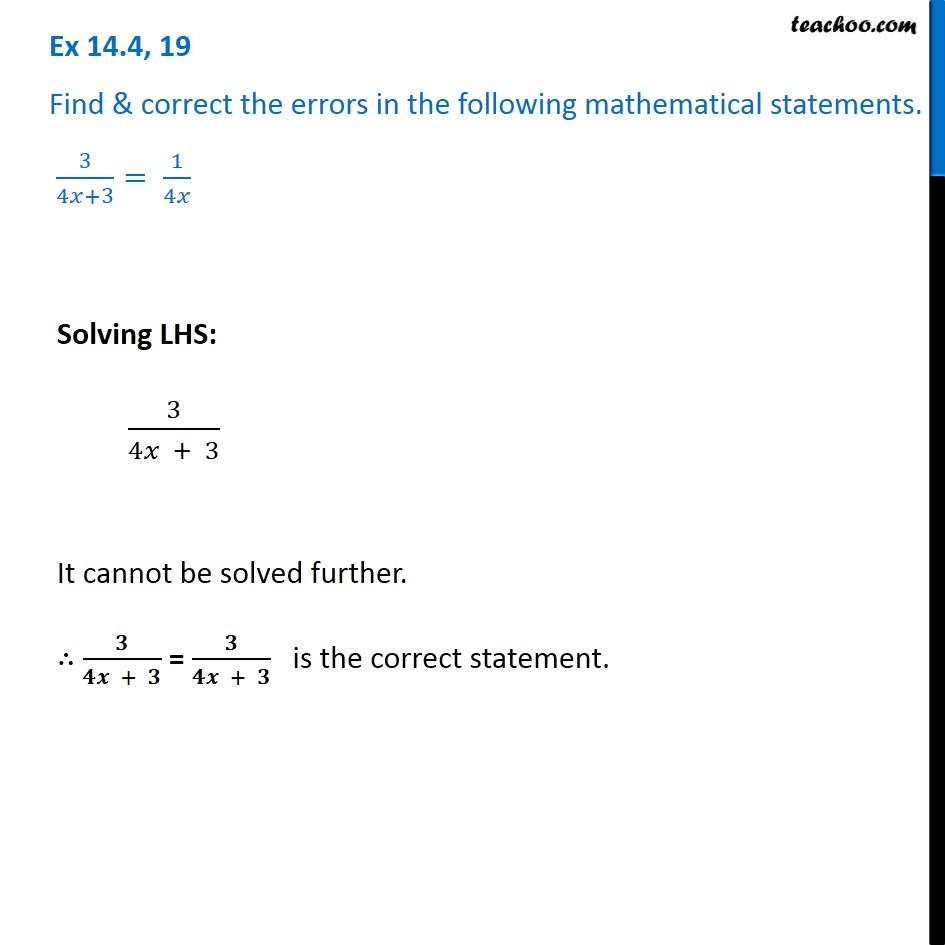1. Chapter 14 Class 8 Factorisation
2. Serial order wise
3. Ex 14.4

Transcript

Ex 14.4, 19 Find & correct the errors in the following mathematical statements. 3/(4𝑥+3)= 1/4𝑥 Solving LHS: 3/(4𝑥 + 3) It cannot be solved further. ∴ 𝟑/(𝟒𝒙 + 𝟑) = 𝟑/(𝟒𝒙 + 𝟑) is the correct statement.

Ex 14.4